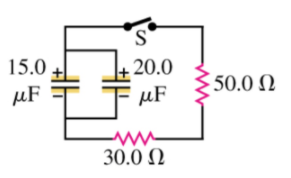# Problem: In the circuit shown in the figure (Figure 1), both capacitors are initially charged to 50.0 V. Part A) How long after closing the switch S will the potential across each capacitor be reduced to 10.0 V?Part B) What will be the current at that time?

###### FREE Expert Solution

The potential across a capacitor in an RC circuit is expressed as:

$\overline{){\mathbf{V}}{\mathbf{=}}{{\mathbf{V}}}_{{\mathbf{0}}}{{\mathbf{e}}}^{\mathbf{-}\frac{\mathbf{t}}{{\mathbf{R}}_{\mathbf{e}\mathbf{q}}{\mathbf{C}}_{\mathbf{e}\mathbf{q}}}}}$, where V is the voltage at a time t, V0 is the initial voltage, t is the time taken for the voltage to reduce from V0 to V, Req is the equivalent resistance, and Ceq is the equivalent capacitance.

Equivalent resistance for resistors in series:

$\overline{){{\mathbf{R}}}_{{\mathbf{eq}}}{\mathbf{=}}{{\mathbf{R}}}_{{\mathbf{1}}}{\mathbf{+}}{{\mathbf{R}}}_{{\mathbf{2}}}{\mathbf{+}}{\mathbf{.}}{\mathbf{.}}{\mathbf{.}}{\mathbf{+}}{{\mathbf{R}}}_{{\mathbf{n}}}}$

For capacitors in parallel, the equivalent capacitance is:

$\overline{){{\mathbf{C}}}_{\mathbf{e}\mathbf{q}}{\mathbf{=}}{{\mathbf{C}}}_{{\mathbf{1}}}{\mathbf{+}}{{\mathbf{C}}}_{{\mathbf{2}}}{\mathbf{+}}{\mathbf{.}}{\mathbf{.}}{\mathbf{.}}{\mathbf{+}}{{\mathbf{C}}}_{{\mathbf{n}}}}$

88% (299 ratings)###### Problem Details

In the circuit shown in the figure (Figure 1), both capacitors are initially charged to 50.0 V.Part A) How long after closing the switch S will the potential across each capacitor be reduced to 10.0 V?

Part B) What will be the current at that time?

Frequently Asked Questions

What scientific concept do you need to know in order to solve this problem?

Our tutors have indicated that to solve this problem you will need to apply the !! Resistor-Capacitor Circuits concept. If you need more !! Resistor-Capacitor Circuits practice, you can also practice !! Resistor-Capacitor Circuits practice problems.